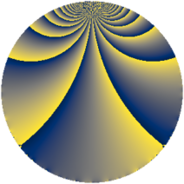# Properties

 Label 216.1.rLevel $216$ Weight $1$ Character orbit 216.r Rep. character $\chi_{216}(43,\cdot)$ Character field $\Q(\zeta_{18})$ Dimension $6$ Newform subspaces $1$ Sturm bound $36$ Trace bound $0$

# Related objects

## Defining parameters

 Level: $$N$$ $$=$$ $$216 = 2^{3} \cdot 3^{3}$$ Weight: $$k$$ $$=$$ $$1$$ Character orbit: $$[\chi]$$ $$=$$ 216.r (of order $$18$$ and degree $$6$$) Character conductor: $$\operatorname{cond}(\chi)$$ $$=$$ $$216$$ Character field: $$\Q(\zeta_{18})$$ Newform subspaces: $$1$$ Sturm bound: $$36$$ Trace bound: $$0$$

## Dimensions

The following table gives the dimensions of various subspaces of $$M_{1}(216, [\chi])$$.

Total New Old
Modular forms 18 18 0
Cusp forms 6 6 0
Eisenstein series 12 12 0

The following table gives the dimensions of subspaces with specified projective image type.

$$D_n$$ $$A_4$$ $$S_4$$ $$A_5$$
Dimension 6 0 0 0

## Trace form

 $$6q - 3q^{8} + O(q^{10})$$ $$6q - 3q^{8} - 3q^{11} - 3q^{12} + 6q^{18} - 3q^{22} - 3q^{27} - 3q^{33} - 3q^{34} + 6q^{38} - 3q^{41} - 3q^{43} + 6q^{51} - 3q^{57} + 6q^{59} - 3q^{64} - 3q^{67} + 6q^{68} + 6q^{76} - 3q^{86} + 6q^{88} + 3q^{89} + 6q^{96} - 3q^{97} - 3q^{98} + O(q^{100})$$

## Decomposition of $$S_{1}^{\mathrm{new}}(216, [\chi])$$ into newform subspaces

Label Dim. $$A$$ Field Image CM RM Traces $q$-expansion
$$a_2$$ $$a_3$$ $$a_5$$ $$a_7$$
216.1.r.a $$6$$ $$0.108$$ $$\Q(\zeta_{18})$$ $$D_{9}$$ $$\Q(\sqrt{-2})$$ None $$0$$ $$0$$ $$0$$ $$0$$ $$q+\zeta_{18}^{2}q^{2}+\zeta_{18}^{8}q^{3}+\zeta_{18}^{4}q^{4}+\cdots$$